JAIC 1990, Volume 29, Number 2, Article 8 (pp. 207 to 220)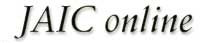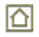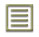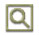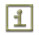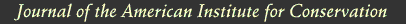JAIC 1990, Volume 29, Number 2, Article 8 (pp. 207 to 220)

### 4 STATISTICAL ANALYSIS

LINEAR REGRESSION analyses for best slope or best straight line were carried out conforming to standard practice (Bennet and Franklin 1954; Youden 1951). Regression coefficients and constants and their standard errors were calculated using built-in functions of Lotus 1-2-3, on an IBM PC AT. The 95% confidence intervals were calculated by multiplying the standard errors of the coefficients with the t-value for the appropriate number of degrees of freedom (Youden 1951, table 1).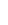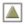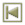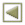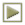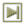Copyright � 1990 American Institute for Conservation of Historic and Artistic Works